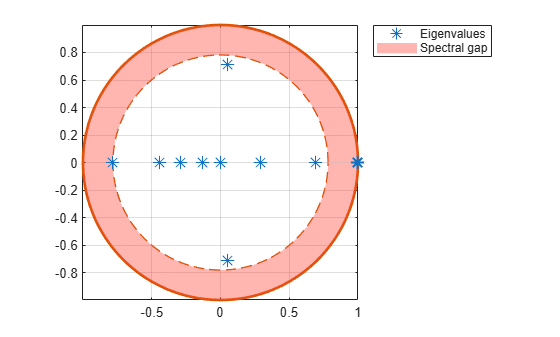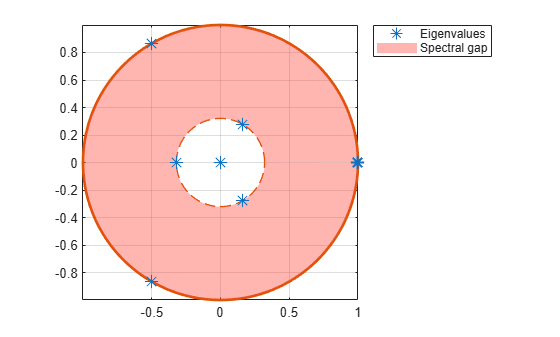# eigplot

Plot Markov chain eigenvalues

## Syntax

``eigplot(mc)``
``eVals = eigplot(mc)``
``eigplot(ax,mc)``
``[eVals,h] = eigplot(___)``

## Description

example

````eigplot(mc)` creates a plot containing the eigenvalues of the transition matrix of the discrete-time Markov chain `mc` on the complex plane. The plot highlights the following: Unit circlePerron-Frobenius eigenvalue at (1,0)Circle of second largest eigenvalue magnitude (SLEM)Spectral gap between the two circles, which determines the mixing time ```

example

``eVals = eigplot(mc)` additionally returns the eigenvalues `eVals` sorted by magnitude.`
``eigplot(ax,mc)` plots on the axes specified by `ax` instead of the current axes (`gca`).`
``` `[eVals,h] = eigplot(___)` additionally returns the handle to the eigenvalue plot using input any of the input arguments in the previous syntaxes. Use `h` to modify properties of the plot after you create it.```

## Examples

collapse all

Create 10-state Markov chains from two random transition matrices, with one transition matrix being more sparse than the other.

```rng(1); % For reproducibility numstates = 10; mc1 = mcmix(numstates,'Zeros',20); mc2 = mcmix(numstates,'Zeros',80); % mc2.P is more sparse than mc1.P```

Plot the eigenvalues of the transition matrices on the separate complex planes.

```figure; eigplot(mc1);``````figure; eigplot(mc2);```The pink disc in the plots show the spectral gap (the difference between the two largest eigenvalue moduli). The spectral gap determines the mixing time of the Markov chain. Large gaps indicate faster mixing, whereas thin gaps indicate slower mixing. Because the spectral gap of `mc1` is thicker than the spectral gap of `mc2`, `mc1` mixes faster than `mc2`.

Consider this theoretical, right-stochastic transition matrix of a stochastic process.

`$P=\left[\begin{array}{ccccccc}0& 0& 1/2& 1/4& 1/4& 0& 0\\ 0& 0& 1/3& 0& 2/3& 0& 0\\ 0& 0& 0& 0& 0& 1/3& 2/3\\ 0& 0& 0& 0& 0& 1/2& 1/2\\ 0& 0& 0& 0& 0& 3/4& 1/4\\ 1/2& 1/2& 0& 0& 0& 0& 0\\ 1/4& 3/4& 0& 0& 0& 0& 0\end{array}\right].$`

Create the Markov chain that is characterized by the transition matrix P.

```P = [ 0 0 1/2 1/4 1/4 0 0 ; 0 0 1/3 0 2/3 0 0 ; 0 0 0 0 0 1/3 2/3; 0 0 0 0 0 1/2 1/2; 0 0 0 0 0 3/4 1/4; 1/2 1/2 0 0 0 0 0 ; 1/4 3/4 0 0 0 0 0 ]; mc = dtmc(P);```

Plot and return the eigenvalues of the transition matrix on the complex plane.

```figure; eVals = eigplot(mc)``````eVals = 7×1 complex -0.5000 + 0.8660i -0.5000 - 0.8660i 1.0000 + 0.0000i -0.3207 + 0.0000i 0.1604 + 0.2777i 0.1604 - 0.2777i -0.0000 + 0.0000i ```

Three eigenvalues have modulus one, which indicates that the period of `mc` is three.

Compute the mixing time of the Markov chain.

`[~,tMix] = asymptotics(mc)`
```tMix = 0.8793 ```

## Input Arguments

collapse all

Discrete-time Markov chain with `NumStates` states and transition matrix `P`, specified as a `dtmc` object. `P` must be fully specified (no `NaN` entries).

Axes on which to plot, specified as an `Axes` object.

By default, `eigplot` plots to the current axes (`gca`).

## Output Arguments

collapse all

Transition matrix eigenvalues sorted by magnitude, returned as a numeric vector.

Handles to plotted graphics objects, returned as a graphics array. `h` contains unique plot identifiers, which you can use to query or modify properties of the plot.

Note

• By the Perron-Frobenius Theorem , a chain with a single recurrent communicating class (a unichain) has exactly one eigenvalue equal to `1` (the Perron-Frobenius eigenvalue), and an accompanying nonnegative left eigenvector that normalizes to a unique stationary distribution. All other eigenvalues have modulus less than or equal to `1`. The inequality is strict unless the recurrent class is periodic. When there is periodicity of period k, there are k eigenvalues on the unit circle at the k roots of unity.

• For an ergodic unichain, any initial distribution converges to the stationary distribution at a rate determined by the second largest eigenvalue modulus (SLEM), μ. The spectral gap, 1 – μ, provides a visual measure, with large gaps (smaller SLEM circles) producing faster convergence. Rates are exponential, with a characteristic time given by

`$tMix=-\frac{1}{\mathrm{log}\left(\mu \right)}.$`

 Gallager, R.G. Stochastic Processes: Theory for Applications. Cambridge, UK: Cambridge University Press, 2013.

 Horn, R., and C. R. Johnson. Matrix Analysis. Cambridge, UK: Cambridge University Press, 1985.

 Seneta, E. Non-negative Matrices and Markov Chains. New York, NY: Springer-Verlag, 1981.